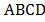### Sample Problem

In the following rectangle, if AD = 9 cm, AB = 6 cm, and ADE = DEBF = CDF then the area of DEF is cm2.#### Solution

Since we cannot find the area of (4) directly, we can calculate the areas of (1) , (2) and (3) first, and then find the area of (4) .The area of

(1) = (2) = (3) + (4) ,

which is equal to a third of the rectangle, 9 × 6 ÷ 3 = 18 cm2.

Since we know the base of AED is 9, 9 × AE ÷ 2 = 18, so

AE = 18 × 2 ÷ 9 = 4 cm.

Similarly, we know the height of DCF = 6, so 6 × CF ÷ 2 = 18, and CF = 18 × 2 ÷ 6 = 6 cm. Since EB = AB = AE and BF = BC-BF, EB = 6 - 4 = 2 cm and BF = 9 - 6 = 3 cm. BE and BF are the height and base of the triangle, so the area of BEF = 2 × 3 ÷ 2 = 3. We know that DEBF = 18, and DEF = DEBF-BEF, so DEF = 18 - 3 = 15 cm2.